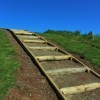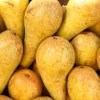# Search by Topic

Filter by: Content type:
Age range:
Challenge level:

### There are 56 results

Broad Topics > Admin > CMEP### Stretching an Integral

##### Age 16 to 18 Challenge Level:

What happens to areas under the graph of $\frac{1}{x}$ when they are stretched?### Trigsy Integrals

##### Age 16 to 18 Challenge Level:

What different ways can you find to calculate these integrals?### Sine Stretching

##### Age 16 to 18 Challenge Level:

Can you find the gradient function of $\sin 3x$. How did you convince yourself?### Slippery Areas

##### Age 16 to 18 Challenge Level:

Can you find the definite integral of a transformed function?### Can You Find... Asymptote Edition

##### Age 16 to 18 Challenge Level:

Can you work out the equation of the function from its graph?### Rotating Derivatives

##### Age 16 to 18 Challenge Level:

By considering a point on a unit circle, can you use geometry to find the derivatives of $\sin x$ and $\cos x$?### Slices of Pi

##### Age 16 to 18 Challenge Level:

How are $\sin x$, $\cos x$ and $\tan x$ related to each other? Can you make sense of the the 'slices'?### Parabella

##### Age 14 to 18 Challenge Level:

Can you prove an algebraic statement using geometric reasoning?### Worth 1000 Words

##### Age 16 to 18 Challenge Level:

Which of the sketches matches the function given?### Muddled Trig

##### Age 16 to 18 Challenge Level:

Can you match all the different trig functions to their graphs?### Translating or Not?

##### Age 16 to 18 Challenge Level:

Can you sketch the graphs of these two reciprocal functions? What are the links between them?### Function Builder 1

##### Age 16 to 18 Challenge Level:

What happens when you combine functions? Can you sketch the results?### Trig Tables

##### Age 16 to 18 Challenge Level:

Can you fill in all the missing values and trig functions to make the tables complete?### Compose

##### Age 16 to 18 Challenge Level:

Can you compose four functions to create the set of functions given?### Two-way Calculus

##### Age 16 to 18 Challenge Level:

Can you find functions with the desired properties to fill in the table?### To Log or Not to Log?

##### Age 16 to 18 Challenge Level:

Which of these logarithmic challenges can you solve?### Log Lattice

##### Age 16 to 18 Challenge Level:

Can you figure out how each log fits into the lattice?### How Fast Does it Grow?

##### Age 16 to 18 Challenge Level:

Exponential functions grow pretty quickly...### Reach for the Stars

##### Age 16 to 18 Challenge Level:

Some graphs grow so quickly you can reach dizzy heights...### $t$ for Tan

##### Age 16 to 18 Challenge Level:

Can you find a geometric proof for some $\tan\theta$ trig identities?### Proving Half-angle Formulae

##### Age 16 to 18 Challenge Level:

Can you find a geometric proof of these half-angle trig identities?### Inspecting Identities

##### Age 16 to 18 Challenge Level:

Using trig identities to help sketch graphs of functions### Trig Countdown

##### Age 16 to 18 Challenge Level:

A game for one or more players in which you make a target value by building a trig expression### Paired Parabolas

##### Age 16 to 18 Challenge Level:

Some parabolas are related to others. How are their equations and graphs connected?### Which Fraction Is Bigger?

##### Age 16 to 18 Challenge Level:

Given two algebraic fractions, how can you decide when each is bigger?### Name That Graph

##### Age 16 to 18 Challenge Level:

How can you work out the equation of a parabola just by looking at key features of its graph?### Between

##### Age 16 to 18 Challenge Level:

If you know some points on a line, can you work out other points in between?### Finding Your Feet

##### Age 16 to 18 Challenge Level:

Problems to help you get started with higher mathematics courses### Designing a Polynomial

##### Age 16 to 18 Challenge Level:

Can you find a polynomial function whose first derivative is equal to the function?### Curvy Cubics

##### Age 16 to 18 Challenge Level:

Use some calculus clues to pin down an equation of a cubic graph.### Integral Chasing

##### Age 16 to 18 Challenge Level:

Can you find the missing constants from these not-quite-so-obvious definite integrals?### Can You Find... Cubic Curves

##### Age 16 to 18 Challenge Level:

Can you find equations for cubic curves that have specific features?### Can You Find... Trigonometric Edition Part 2

##### Age 16 to 18 Challenge Level:

Can you find trig graphs to satisfy a variety of conditions?### Which Parabola?

##### Age 16 to 18 Challenge Level:

Can you work out the equations of all these mixed up parabolas?### Slippery Slopes

##### Age 16 to 18 Challenge Level:

When you transform a function, what can you work out about the gradient?### Name That Graph Again

##### Age 16 to 18 Challenge Level:

If you know some information about a parabola, can you work out its equation?### Irrational Constructions

##### Age 16 to 18 Challenge Level:

What questions does the spiral construction prompt you to ask?### Nested Surds

##### Age 16 to 18 Challenge Level:

Can you find values that make these surd statements true?### Irrational Roots

##### Age 16 to 18 Challenge Level:

You're used to working with quadratics with integer roots, but what about when the roots are irrational?### Staircase Sequences

##### Age 16 to 18 Challenge Level:

Can you make sense of these unusual fraction sequences?### Have a Sine

##### Age 16 to 18 Challenge Level:

There's much more to trigonometry than sin, cos and tan...### Equation or Identity (2)

##### Age 16 to 18 Challenge Level:

Here are some more triangle equations. Which are always true?### Equation or Identity (1)

##### Age 16 to 18 Challenge Level:

Which of these equations concerning the angles of triangles are always true?### Can You Find... Trigonometry Edition

##### Age 16 to 18 Challenge Level:

What graphs can you make by transforming sine, cosine and tangent graphs?### The Circle of Apollonius... Coordinate Edition

##### Age 16 to 18 Challenge Level:

Can you sketch and then find an equation for the locus of a point based on its distance from two fixed points?### Finding Circles

##### Age 16 to 18 Challenge Level:

Can you find the centre and equation of a circle given a number of points on the circle? When is it possible and when is it not?### Quadrature of the Lunes

##### Age 16 to 18 Challenge Level:

A lune is the area left when part of a circle is cut off by another circle. Can you work out the area?### The Quintessential Proof

##### Age 16 to 18 Challenge Level:

In this resource, the aim is to understand a fundamental proof of Pythagoras's Theorem.### Transformation ... or Not?

##### Age 16 to 18 Challenge Level:

This graph looks like a transformation of a familiar function...### A Tangent Is ...

##### Age 16 to 18 Challenge Level:

What do we REALLY mean when we talk about a tangent to a curve?